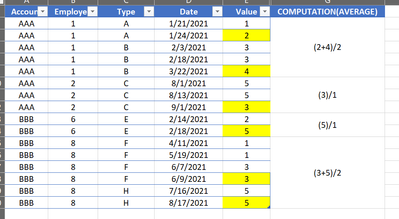cancel
Showing results for
Did you mean:Anonymous
Not applicable

## MEASURE CALCULATING THE AVERAGE OF ALL THE VALUES FROM MAX DATES BY MULTPLE COLUMNS

Hi Guys,

Newbie here,

Can you help me create a measure that will calculate the average of all the last values from a certain column based on a date with a group by function?

For example.

Highlighted in YELLOW are the values from the MAX of date column based on ACCOUNT, EMPLOYEE, and TYPE.

RAW TABLEDESIRED OUTPUT:I hope I clearly explained what am I trying to accomplish. Let me know for any questions.

2 ACCEPTED SOLUTIONSCommunity Support

Hi @Anonymous ,

First create a calculated column as below:

``````_check =
VAR _maxdate =
MAXX (
FILTER (
ALL ( 'Table' ),
'Table'[Account] = EARLIER ( 'Table'[Account] )
&& 'Table'[Employee] = EARLIER ( 'Table'[Employee] )
&& 'Table'[Type] = EARLIER ( 'Table'[Type] )
),
[Date]
)
RETURN
IF ( 'Table'[Date] = _maxdate, 1, BLANK () )
``````

Then create a measure as below:

``````Average =
VAR _sum =
SUMX (
FILTER (
ALL ( 'Table' ),
'Table'[Account] = MAX ( 'Table'[Account] )
&& 'Table'[Employee] = MAX ( 'Table'[Employee] )
&& 'Table'[_check] = 1
),
'Table'[Value]
)
VAR _count =
CALCULATE (
COUNT ( 'Table'[Account] ),
FILTER (
ALL ( 'Table' ),
'Table'[Account] = MAX ( 'Table'[Account] )
&& 'Table'[Employee] = MAX ( 'Table'[Employee] )
&& 'Table'[_check] = 1
)
)
RETURN
DIVIDE ( _sum, _count )
``````

And you will see:For the related .pbix file,pls see attached.

Best Regards,
KellySuper User

Change All Function with AllSELECTED hope it will works

5 REPLIES 5Super User

@Anonymous , Try a measure like

calculate(averageX(Table, LASTNONBLANK(Table[Date], Max(Table[Value]))), filter(allselected(Table), [ACCOUNT] = max(Table[Account]) && [EMPLOYEE] = max(Table[EMPLOYEE])
&& [TYPE] = max(Table[Type])))Anonymous
Not applicable

Hi @amitchandak ,

Thank you for your response. However, the result is different from what I've expected. Can you check if there is something that I did wrong?

Please see the screenshot below for the result of my query.Community Support

Hi @Anonymous ,

First create a calculated column as below:

``````_check =
VAR _maxdate =
MAXX (
FILTER (
ALL ( 'Table' ),
'Table'[Account] = EARLIER ( 'Table'[Account] )
&& 'Table'[Employee] = EARLIER ( 'Table'[Employee] )
&& 'Table'[Type] = EARLIER ( 'Table'[Type] )
),
[Date]
)
RETURN
IF ( 'Table'[Date] = _maxdate, 1, BLANK () )
``````

Then create a measure as below:

``````Average =
VAR _sum =
SUMX (
FILTER (
ALL ( 'Table' ),
'Table'[Account] = MAX ( 'Table'[Account] )
&& 'Table'[Employee] = MAX ( 'Table'[Employee] )
&& 'Table'[_check] = 1
),
'Table'[Value]
)
VAR _count =
CALCULATE (
COUNT ( 'Table'[Account] ),
FILTER (
ALL ( 'Table' ),
'Table'[Account] = MAX ( 'Table'[Account] )
&& 'Table'[Employee] = MAX ( 'Table'[Employee] )
&& 'Table'[_check] = 1
)
)
RETURN
DIVIDE ( _sum, _count )
``````

And you will see:For the related .pbix file,pls see attached.

Best Regards,
KellyAnonymous
Not applicable

Thank you for this solution. It got the correct output that I wanted.

However, what if I have a TYPE slicer into the dashboard? The result is still the same which must be only the selected filters only will be computed in the measure.

Thank you for your assistance on this.Super User

Change All Function with AllSELECTED hope it will works